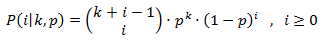class template
<random>

# std::negative_binomial_distribution

`template <class IntType = int> class negative_binomial_distribution;`
Negative binomial distribution
Random number distribution that produces integers according to a negative binomial discrete distribution (also known as Pascal distribution), which is described by the following probability mass function:This distribution produces random integers where each value represents the number of successful trials before k unsuccessful trials happen in a sequence of trials, each with a probability of success equal to p.

To produce a random value following this distribution, call its member function operator().

### Template parameters

IntType
An integer type. Aliased as member type result_type.
By default, this is int.

### Member types

The following aliases are member types of negative_binomial_distribution:

member typedefinitionnotes
result_typeThe first template parameter (IntType)The type of the numbers generated (defaults to int)
param_typenot specifiedThe type returned by member param.

### Example

 ``12345678910111213141516171819202122232425`` ``````// negative_binomial_distribution #include #include int main() { const int nrolls = 10000; // number of experiments const int nstars = 100; // maximum number of stars to distribute std::default_random_engine generator; std::negative_binomial_distribution distribution(3,0.5); int p={}; for (int i=0; i

Possible output:
 ```negative_binomial_distribution (k=3,p=0.5): 0: ************ 1: ******************* 2: ***************** 3: **************** 4: *********** 5: ******* 6: ***** 7: *** 8: ** 9: * ```